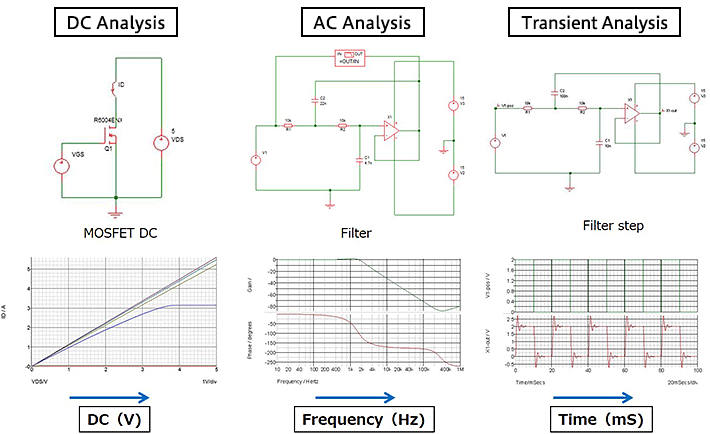# Types of SPICE simulation: DC Analysis, AC Analysis, Transient Analysis

2018.10.25

・SPICE-based simulators are provided with functions for DC analysis, AC analysis, transient analysis, Monte Carlo analysis, S parameter and Fourier analysis, and noise analysis, among others.

・Nearly all simulator software packages are provided with functions for DC analysis, AC analysis, transient analysis, and Monte Carlo analysis.

Here we describe representative analytical functions and present a summary to indicate the capabilities of a SPICE-based simulator.

## Types of SPICE Simulation

The representative functions of SPICE-based simulators are summarized below. The highlighted DC analysis, AC analysis, transient analysis, and Monte Carlo functions are well-known analysis functions that are included in nearly all simulator software packages. These four types of function are explained in some detail. In this article, we discuss DC analysis, AC analysis, and transient analysis.

Analysis Method Function/Characteristic
DC analysis Analysis of static characteristics. (All DC characteristics of the data sheet)
AC analysis Analysis of frequency characteristics. (Capacitance, gain-phase)
Transient analysis Time response analysis. (Oscilloscope)
Monte Carlo Simulations that reflect variation in circuit elements.
*Variation must be described in the model. The description format differs with the software.
S-parameter High-frequency characteristic analysis.
Fourier analysis High-frequency analysis and signal distortion analysis.
Noise analysis Noise analysis at measurement points.
*If noise is not included in the model, noise does not occur.

### SPICE Simulations: DC Analysis, AC Analysis, Transient Analysis

These analyses are the most basic fundamentals of device and circuit analysis, and so perhaps need not be explained here, but examples are shown below.DC analysis is analysis of static characteristics. In the above example, the change in ID with VDS for a MOSFET is simulated. In general, values stipulated on the data sheet of a transistor, IC, or other device will include DC characteristics and AC characteristics. DC analysis is capable of simulation of all the DC characteristics in the data sheet.

AC analysis mainly addresses characteristics relating to frequency. Representative quantities include analysis of the impedance as a function of frequency and of the gain-phase characteristic. The above example simulates the gain and phase characteristics versus frequency for a filter circuit.

Transient analysis is analysis of the time response. The above example shows the step response of a filter, but this method is also often used in evaluation of the load transient response of switching power supplies. Transient analysis typically involves using an oscilloscope to observe waveforms.

The various simulators are provided with diverse functions for performing these analyses, such as current/voltage sources, function generators (oscillators), and current or voltage measurement; measurement results can be plotted in graphs.

In the next article, we will explain the remaining analysis type, Monte Carlo analysis.